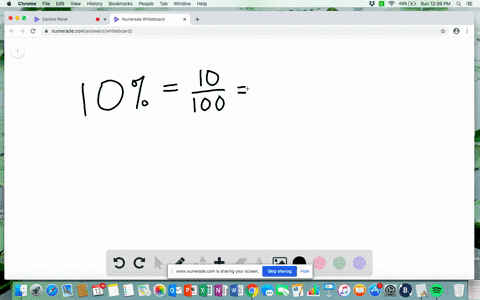Enroll in one of our FREE online STEM summer camps. Space is limited so join now!View Summer Courses### In the following exercises, convert each percent …

01:21Need more help? Fill out this quick form to get professional live tutoring.

Get live tutoring
Problem 45

In the following exercises, convert each percent to
(a) a simplified fraction and (b) a decimal

A couple plans to have two children. The probability they will have two girls is 25$\% .$

\begin{array}{l}{\text { a. As a fraction: }} \\ {\qquad \begin{aligned} 25 \% &=\frac{25}{100} \\ \text { b. As a decimal: } & \\ 25 \% &=25 \\ &=0.25 \end{aligned}}\end{array}

## Discussion

You must be signed in to discuss.

## Video Transcript

So in this problem we have 25% and we need to turn it into a fraction and then into a decimal. So first, let's think about the word percent. It's two parts with her meaning out of and sent meaning 100 out of 100 so we can go ahead and write This 25% has 25 over 100 we're going to use this fraction later to help us chart into a decimal. But for now, let's go ahead and simplify this fraction. So in 25 over 100 both the top and the bottom are divisible by five. And when we do that, we're going to get five over 20. Well, now we can do it again. Divide both the top and the bottom by five, and we're going to get one over for that is the most simplified. We can make this fraction. So the answer for part A is 1/4. Now let's move into the decimal. We have 25 over 100 we would turn it into a decimal. Well, we know that this decimal has two zeros in the you know, this fraction is two zeros in the denominator so our fraction can look like this. Okay, so we go ahead and write it out. We have 20 plus five over 100. Well, there's nothing in this. There's nothing in the, uh, in the ones place so we could go ahead and put a zero there. And now, in the tense place, we have 20. So two of those tens that's a two there and five over 100 makes five in the hundreds place. So our answer for part B is 0.25